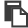【单选题】肋下横切或斜切时,正确构成肝左叶“工”字形结构的血管是 ( )
A.

B.

C.

D.手机使用分享复制链接新浪微博分享QQ微信扫一扫反馈复制

【单选题】应采用什么途径来获得肝右叶下缘角( )
A.

B.

C.

D.

E.

【单选题】肝左外叶、左内叶的分界标志为
A.

B.

C.

D.

【单选题】不与肝右叶相邻的器官是
A.

B.

C.

D.

E.

【单选题】肝叶切除病人的术后护理错误的是
A.

B.

C.

D.

E.

【单选题】肝的分叶不包括
A.

B.

C.

D.

【单选题】不属于肝的分叶的是
A.

B.

C.

D.

【单选题】肝叶切除患者的术后护理，下列错误的是（）
A.

B.

C.

D.

E.

【简答题】的下面借 "H" 形沟可分为 肝左叶 、 肝右叶 、 方叶 和 尾状叶 四个叶。
【单选题】肝右叶下缘角正常应小于( )
A.
30°
B.
45°
C.
50°
D.
60°
E.
75°
【单选题】肝的分叶不包括
A.

B.

C.

D.

【单选题】划分左、右肝叶的解剖标志是
A.

B.

C.

D.

E.

【单选题】正确构成肝左叶“工”字形结构的血管是
A.

B.

C.

D.

E.

【判断题】肝左静脉是肝左内叶和肝外叶的分界标志
A.

B.

【单选题】患者男性，50岁，因肝右叶肝癌而行肝叶切除术，患者的肝发生的变化是
A.

B.

C.

D.

【单选题】在肝膈面, 区分肝右叶和肝左叶的分界标志是( )
A.

B.

C.

D.

E.

【单选题】位于肝左外叶,主要治疗措施为
A.

B.

C.

D.

E.

【单选题】肝叶切除患者的术后护理错误的是
A.

B.

C.

D.

E.

【单选题】肝叶切除患者的术后护理错误的是
A.

B.

C.

D.

E.

【单选题】不属于肝的分叶的是
A.

B.

C.

D.

【单选题】肝左叶与尾状叶的分界标志是:( )
A.

B.

C.

D.

E.

【单选题】肝右叶、左叶的分界标志
A.

B.

C.

D.

【单选题】肝右叶、左叶的分界标志( )
A.

B.

C.

D.

【单选题】患者,女,48岁,因肝癌需行肝左叶切除术。关于肝的分叶的描述,正确的是
A.

B.

C.

D.

E.

【单选题】肝叶切除患者术后的护理,下列哪项是错误的
A.

B.

C.

D.

E.

【单选题】关于肝的分叶、分段,下列哪项的描述是错误的:
A.

B.

C.

D.

E.

【单选题】男性,50岁,因肝右叶肝癌而行肝叶切除术,患者的肝脏发生的变化是
A.

B.

C.

D.

E.

【单选题】划分左、右肝叶的解剖标志是 ( )
A.

B.

C.

D.

E.

【单选题】男性,50岁,因肝右叶肝癌而行肝叶切除术,患者的肝脏发生的变化是
A.

B.

C.

D.

E.

【单选题】肝叶切除术适用于（）
A.

B.

C.

D.

E.

【简答题】肝右静脉走行于右叶间裂内，是下面哪一项解剖结构的分界标志：()。 A.肝左内叶、左外叶 B.肝右叶、肝左叶 C.肝左叶、尾状叶 D.肝右叶、尾状叶 E.肝右前叶、肝右后叶
【单选题】哪根韧带把肝脏分为肝左叶和肝右叶
A.

B.

C.

D.

【单选题】以下哪种途径是主要探测肝左叶的( )
A.

B.

C.

D.

E.

【单选题】肝左叶下缘角正常应小于( )
A.
25°
B.
30°
C.
45°
D.
50°
E.
60°
【单选题】肝叶切除患者的术后护理错误的是
A.

B.

C.

D.

E.

【单选题】下列结构不与肝右叶下面毗邻的是
A.

B.

C.

D.

E.

【单选题】划分左、右肝叶的解剖标志是
A.

B.

C.

D.

【单选题】肝左叶与尾状叶的分界标志是
A.

B.

C.

D.

E.

【单选题】肝叶切除病人的术后护理错误的是
A.

B.

C.

D.

E.

【单选题】应采用什么途径来获得肝左叶下缘角( )
A.

B.

C.

D.

【简答题】肝叶切除适合于
【简答题】如何预防肝叶切除病人出现肝昏迷?12．  如何预防肝叶切除病人出现肝昏迷?
【单选题】肝右叶、左叶的分界标志
A.

B.

C.

D.

E.

【单选题】肝叶切除病人的术后护理错误的是
A.

B.

C.

D.

E.

【单选题】正确构成肝左叶“工”字形结构的血管是
A.

B.

C.

D.

E.

【单选题】不与肝右叶毗邻的结构是
A.

B.

C.

D.

E.

【单选题】肝右叶、左叶的分界标志
A.

B.

C.

D.

【单选题】肝叶切除患者的术后护理措施中错误的是
A.

B.

C.

D.

E.

【单选题】肝桥连接的肝叶
A.

B.

C.

D.

E.

【单选题】肝右叶间裂内有
A.

B.

C.

D.

E.

【简答题】肝脏触诊时沿____触诊肝右叶,沿____触诊肝左叶。触诊起点分别位于右下腹____水平和前正中线上____ 水平 。